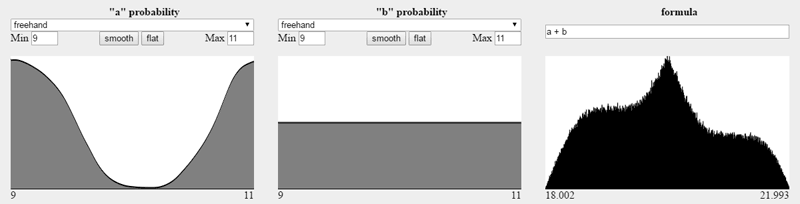"a" probability "b" probability formula

Given two measurements with probability distributions of possible values, and an arbitrary formula combining them, what does the distribution of results look like?

Initially we see two measurements that are uniformly distributed between 9 and 11 (i.e. 10 ± 1), multiplied together. The result is a range of values between 81 and 121, centered around 100.

Try adjusting the "n" slider to control an offset on one of them. Try changing the formula. Drag your mouse over the grey areas below "a" and "b" to adjust the probability of various values. Are the results what you expected?

Here's an example that really surprised me. Can you explain it?(Sketch this yourself with the formula `a + b/n` and play with the value of `n`. Or, try playing with the min/max values for `a` and `b` and see what happens.)